• 在MATLAB软件中，采用互信息法计算时间序列的延迟时间。
• 且改主函数含有各个子函数，整个函数是完整的。这个是将网上的互信息法进行改进，适用性更好。
• MATLAB程序互信息法求混沌时间序列延迟时间，属于自己按照公式自编，亲测可用。两个函数需要调用一下，可自己修改一下。
• python-互信息法求延时嵌入重构参数τ互信息法python代码 互信息法 互信息法是一种求延时嵌入相空间重构参数延时τ的有效方法，本文利用python实现这种算法，仅供参考。 python代码 def pmf_get(datalist,n): ...
python-互信息法求延时嵌入重构参数τ互信息法python代码
互信息法
互信息法是一种求延时嵌入相空间重构参数延时τ的有效方法，本文利用python实现这种算法，仅供参考。
python代码
def pmf_get(datalist,n):
[occur, bin] = np.histogram(datalist, bins=n)
pmf = occur[0: n] / len(datalist)
return pmf, bin

def jointProb_get(jointdata, n):
jointProbs, edges = np.histogramdd(jointdata, bins=[n, n])
jointProbs /= jointProbs.sum()
return jointProbs, edges

def info_entropy_single(data_list, bin, pmf):
H_s = 0
for i in range(len(data_list)):
for j in range(n):
if bin[j] <= data_list[i] < bin[j + 1]:
H_s += (-pmf[j] * np.log2(pmf[j]))
return H_s

def mutual_information(data_list, taustep=1, n=50):
"""
:param data_list: list 时间序列数据
:param taustep: int tau间隔
:param n: int 分箱数
:return: int 最优延迟数
"""
I_sq_list = []
tau = 0
while 1:
print(tau)
select_list0 = np.arange(0, len(data_list) - tau)
select_list1 = select_list0 + tau
x0 = [data_list[i] for i in select_list0]
x1 = [data_list[i] for i in select_list1]
jointdata = np.array([[x0[i], x1[i]] for i in range(len(x0))])

pmf0, bin0 = pmf_get(x0, n)
pmf1, bin1 = pmf_get(x1, n)
jointProbs, edges = jointProb_get(jointdata, n)

H_sq = 0
for data in jointdata:
for i in range(n):
for j in range(n):
if edges[i] <= data < edges[i + 1] and edges[j] <= data < edges[j + 1]:
H_sq = H_sq + (jointProbs[i, j] * np.log2((jointProbs[i, j]) / (pmf0[i] * pmf1[j])))
I_sq_list.append(H_sq)
if len(I_sq_list) > 2:
if I_sq_list[int(tau/taustep)] - I_sq_list[int(tau/taustep-1)] > 0 and I_sq_list[int(tau/taustep-1)] - I_sq_list[int(tau/taustep-2)] < 0:
tau_selected = tau - 1
break
tau += taustep
return tau_selected
if __name__ == "__main__":
x = np.arange(0,10000, 1)
data_preprocess = np.sin(x)
tau = mutual_information(data_preprocess, tau_step, n=20)




展开全文信号处理 python
• 互信息法求最小嵌入维数，网上下载的，直接输入数据可用
• 本文提出了一种新的红外与可见光图像的配准算法,该方法基于图像的小波变换与互信息最大化 完成图像的配准过程。首先通过搜索小波模极大提取图像感兴趣区域(ROI) ,完成图像预处理,并采用仿射 变换,建立图像变换模型;...
• 3.互信息法 4 卡方检验 5.1嵌入的方法： 基于L1范数：使用带惩罚项的基模型，除了筛选出特征外，同时也进行了降维 5.2嵌入的方法： 基于树模型的特征选择：树模型中GBDT也可用来作为基模型进行特征选择，使用...
最近处理数据的时候尝试了一下特征选择，在此记录一下常用的方法，以便日后查阅：
先贴一下原始数据：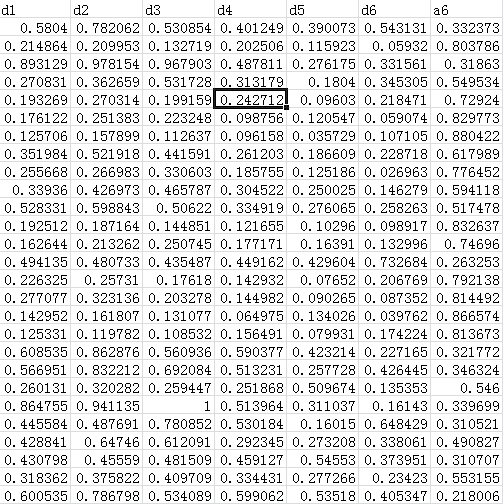1.RFE特征选择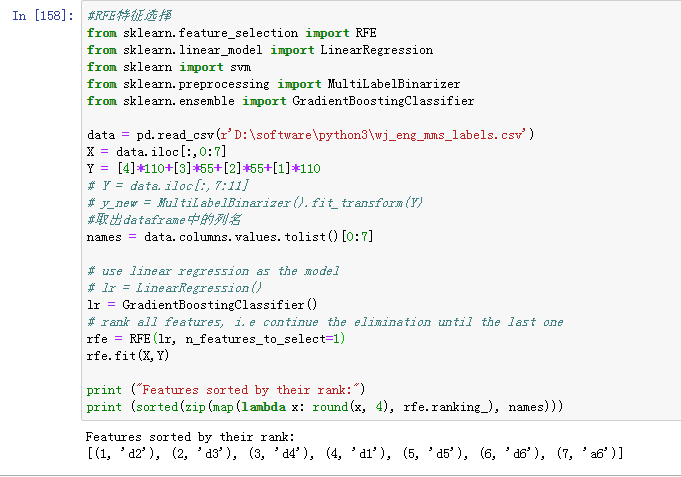2.方差分析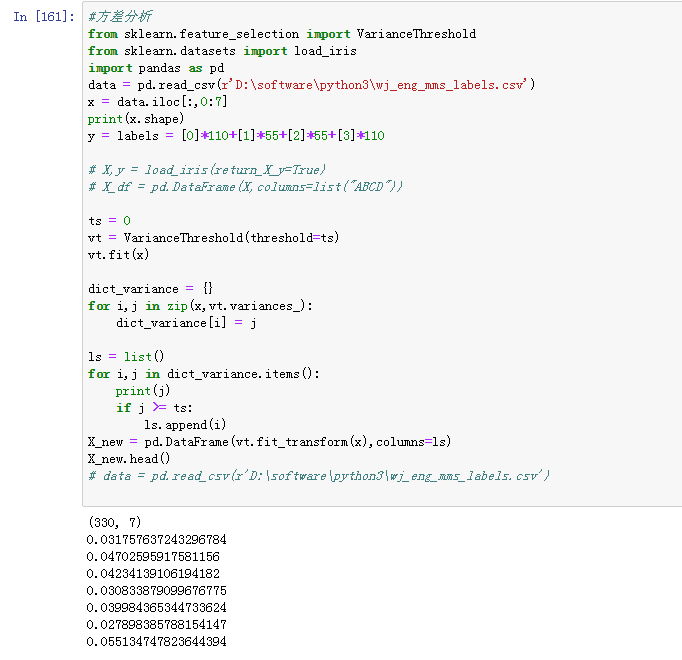3.互信息法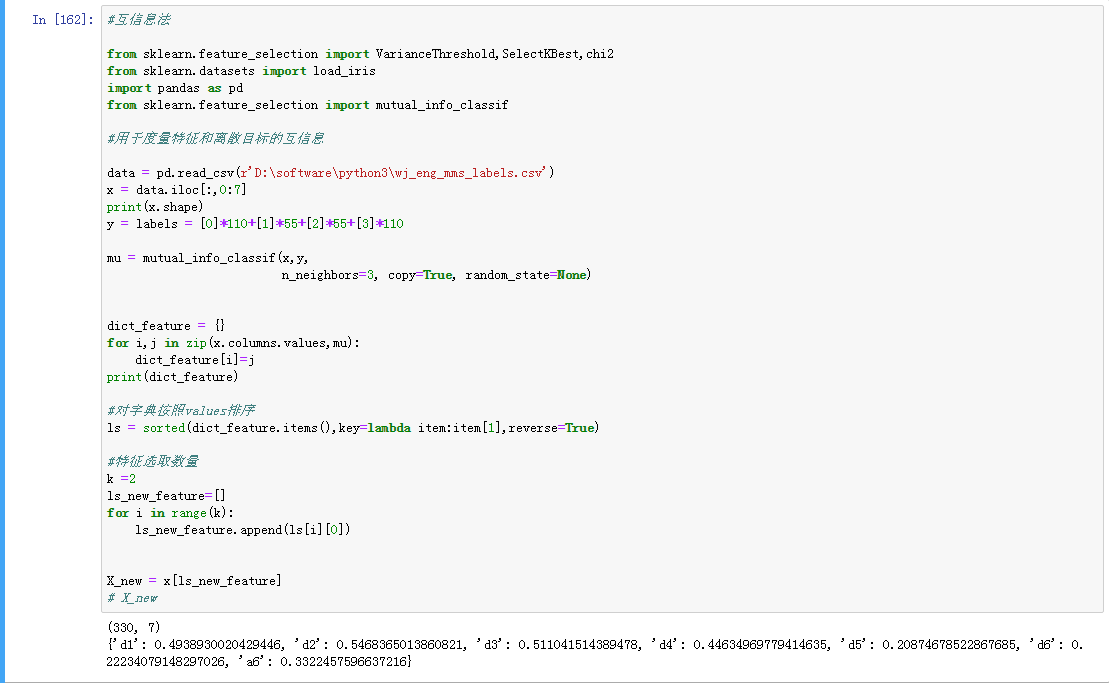4 卡方检验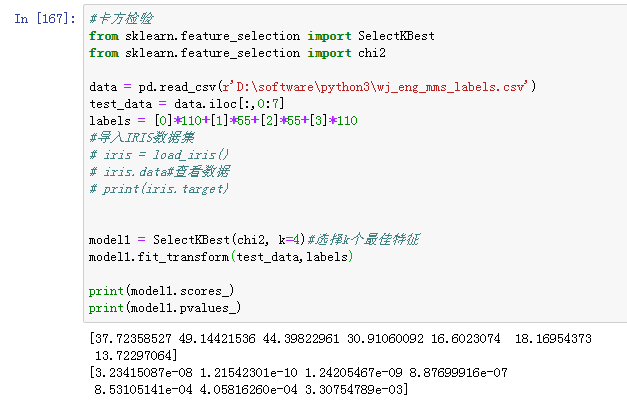5.1嵌入的方法：
基于L1范数：使用带惩罚项的基模型，除了筛选出特征外，同时也进行了降维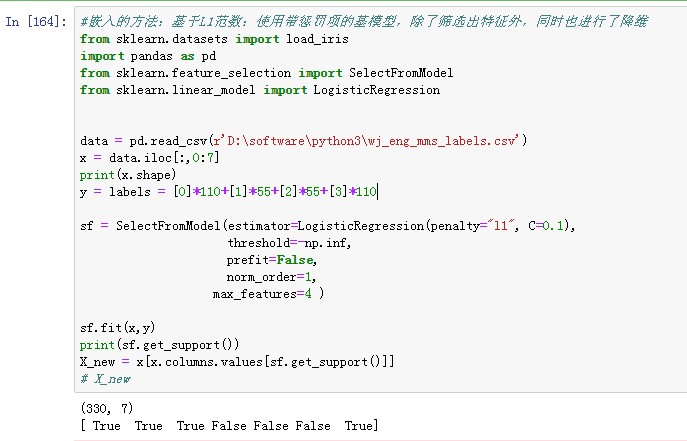5.2嵌入的方法：
基于树模型的特征选择：树模型中GBDT也可用来作为基模型进行特征选择，使用feature_selection库的SelectFromModel类结合GBDT模型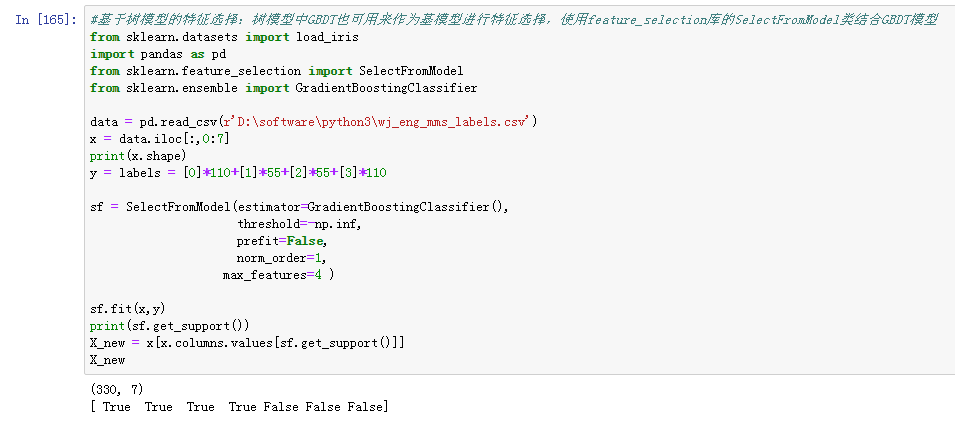展开全文特征选择 FRE 卡方检验 方差分析
• 特征选择主要从两个方面入手：特征是否发散：特征发散说明特征的方差大，能够根据取值的差异化度量目标信息.特征与目标相关性：优先选取与目标高度相关性的.对于特征选择，有时候我们需要考虑分类变量和连续变量的...
特征选择主要从两个方面入手：特征是否发散：特征发散说明特征的方差大，能够根据取值的差异化度量目标信息.特征与目标相关性：优先选取与目标高度相关性的.对于特征选择，有时候我们需要考虑分类变量和连续变量的不同.1.过滤法：按照发散性或者相关性对各个特征进行评分，设定阈值或者待选择阈值的个数选择特征方差选择法：建议作为数值特征的筛选方法计算各个特征的方差，然后根据阈值，选择方差大于阈值的特征from sklearn.feature_selection importVarianceThresholdfrom sklearn.datasets importload_irisimportpandas as pdX,y= load_iris(return_X_y=True)X_df= pd.DataFrame(X,columns=list("ABCD"))#建议作为数值特征的筛选方法，对于分类特征可以考虑每个类别的占比问题ts = 0.5vt= VarianceThreshold(threshold=ts)vt.fit(X_df)#查看各个特征的方差dict_variance ={}for i,j inzip(X_df.columns.values,vt.variances_):dict_variance[i]=j#获取保留了的特征的特征名ls=list()for i,j indict_variance.items():if j >=ts:ls.append(i)X_new= pd.DataFrame(vt.fit_transform(X_df),columns=ls)卡方检验：建议作为分类问题的分类变量的筛选方法经典的卡方检验是检验定性自变量对定性因变量的相关性。假设自变量有N种取值，因变量有M种取值，考虑自变量等于i且因变量等于j的样本频数的观察值与期望的差距，构建统计量：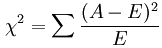from sklearn.feature_selection importVarianceThreshold,SelectKBest,chi2from sklearn.datasets importload_irisimportpandas as pdX,y= load_iris(return_X_y=True)X_df= pd.DataFrame(X,columns=list("ABCD"))(chi2,pval)=chi2(X_df,y)dict_feature={}for i,j inzip(X_df.columns.values,chi2):dict_feature[i]=j#对字典按照values排序ls = sorted(dict_feature.items(),key=lambda item:item,reverse=True)#特征选取数量k =2ls_new_feature=[]for i inrange(k):ls_new_feature.append(ls[i])X_new= X_df[ls_new_feature]互信息法：建议作为分类问题的分类变量的筛选方法经典的互信息也是评价定性自变量对定性因变量的相关性的，为了处理定量数据，最大信息系数法被提出，互信息计算公式如下：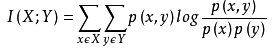from sklearn.feature_selection importVarianceThreshold,SelectKBest,chi2from sklearn.datasets importload_irisimportpandas as pdfrom sklearn.feature_selection importmutual_info_classif#用于度量特征和离散目标的互信息X,y = load_iris(return_X_y=True)X_df= pd.DataFrame(X,columns=list("ABCD"))feature_cat= ["A","D"]discrete_features=[]feature=X_df.columns.values.tolist()for k infeature_cat:if k infeature:discrete_features.append(feature.index(k))mu= mutual_info_classif(X_df,y,discrete_features=discrete_features,n_neighbors=3, copy=True, random_state=None)dict_feature={}for i,j inzip(X_df.columns.values,mu):dict_feature[i]=j#对字典按照values排序ls = sorted(dict_feature.items(),key=lambda item:item,reverse=True)#特征选取数量k =2ls_new_feature=[]for i inrange(k):ls_new_feature.append(ls[i])X_new= X_df[ls_new_feature]from sklearn.feature_selection importVarianceThreshold,SelectKBest,chi2from sklearn.datasets importload_irisimportpandas as pdfrom sklearn.feature_selection importmutual_info_classif,mutual_info_regression#用于度量特征和连续目标的互信息X,y = load_iris(return_X_y=True)X_df= pd.DataFrame(X,columns=list("ABCD"))feature_cat= ["A","D"]discrete_features=[]feature=X_df.columns.values.tolist()for k infeature_cat:if k infeature:discrete_features.append(feature.index(k))mu= mutual_info_regression(X_df,y,discrete_features=discrete_features,n_neighbors=3, copy=True, random_state=None)dict_feature={}for i,j inzip(X_df.columns.values,mu):dict_feature[i]=j#对字典按照values排序ls = sorted(dict_feature.items(),key=lambda item:item,reverse=True)#特征选取数量k =2ls_new_feature=[]for i inrange(k):ls_new_feature.append(ls[i])X_new= X_df[ls_new_feature]2.包装法递归特征消除法：用一个基模型来进行多轮训练，每轮训练后，消除若干权值系数的特征，再基于新的特征集进行下一轮训练from sklearn.datasets importload_irisimportpandas as pdfrom sklearn.feature_selection importRFE,RFECVfrom sklearn.ensemble importRandomForestClassifierX,y= load_iris(return_X_y=True)X_df= pd.DataFrame(X,columns=list("ABCD"))refCV= RFECV(estimator=RandomForestClassifier(),step=0.5,cv=5,scoring=None,n_jobs=-1)refCV.fit(X_df,y)#保留特征的数量refCV.n_features_#保留特征的False、True标记refCV.support_feature_new=X_df.columns.values[refCV.support_]#交叉验证分数refCV.grid_scores_3.嵌入的方法基于L1范数：使用带惩罚项的基模型，除了筛选出特征外，同时也进行了降维from sklearn.datasets importload_irisimportpandas as pdfrom sklearn.feature_selection importSelectFromModelfrom sklearn.linear_model importLogisticRegressionX,y= load_iris(return_X_y=True)X_df= pd.DataFrame(X,columns=list("ABCD"))sf= SelectFromModel(estimator=LogisticRegression(penalty="l1", C=0.1),threshold=None,prefit=False,norm_order=1)sf.fit(X_df,y)X_new= X_df[X_df.columns.values[sf.get_support()]]基于树模型的特征选择法：树模型中GBDT也可用来作为基模型进行特征选择，使用feature_selection库的SelectFromModel类结合GBDT模型from sklearn.datasets importload_irisimportpandas as pdfrom sklearn.feature_selection importSelectFromModelfrom sklearn.ensemble importGradientBoostingClassifierX,y= load_iris(return_X_y=True)X_df= pd.DataFrame(X,columns=list("ABCD"))sf= SelectFromModel(estimator=GradientBoostingClassifier(),threshold=None,prefit=False,norm_order=1)sf.fit(X_df,y)X_new= X_df[X_df.columns.values[sf.get_support()]]
展开全文• 3.1.2 相关性过滤方差挑选完毕之后，我们...在sklearn当中，我们有三种常用的方法来评判特征与标签之间的相关性：卡方，F检验，互信息3.1.2.1 卡方过滤卡方过滤是专门针对离散型标签(即分类问题)的相关性过滤。卡方...
3.1.2 相关性过滤方差挑选完毕之后，我们就要考虑下一个问题：相关性了。我们希望选出与标签相关且有意义的特征，因为这样的特征能够为我们提供大量信息。如果特征与标签无关，那只会白白浪费我们的计算内存，可能还会给模型带来噪音。在sklearn当中，我们有三种常用的方法来评判特征与标签之间的相关性：卡方，F检验，互信息3.1.2.1 卡方过滤卡方过滤是专门针对离散型标签(即分类问题)的相关性过滤。卡方检验类feature_selection.chi2计算每个非负特征和标签之间的卡方统计量，并依照卡方统计量由高到低为特征排名。再结合feature_selection.SelectKBest这个可以输入”评分标准“来选出前K个分数最高的特征的类，我们可以借此除去最可能独立于标签，与我们分类目的无关的特征。另外，如果卡方检验检测到某个特征中所有的值都相同，会提示我们使用方差先进行方差过滤。并且，刚才我们已经验证过，当我们使用方差过滤筛选掉一半的特征后，模型的表现时提升的。因此在这里，我们使用threshold=中位数时完成的方差过滤的数据来做卡方检验(如果方差过滤后模型的表现反而降低了，那我们就不会使用方差过滤后的数据，而是使用原数据)：from sklearn.ensemble import RandomForestClassifier as RFCfrom sklearn.model_selection import cross_val_scorefrom sklearn.feature_selection import SelectKBestfrom sklearn.feature_selection import chi2#假设在这里我一直我需要300个特征X_fschi = SelectKBest(chi2, k=300).fit_transform(X_fsvar, y)X_fschi.shape验证一下模型的效果如何：cross_val_score(RFC(n_estimators=10,random_state=0),X_fschi,y,cv=5).mean()可以看出，模型的效果降低了，这说明我们在设定k=300的时候删除了与模型相关且有效的特征，我们的K值设置得太小，要么我们需要调整K值，要么我们必须放弃相关性过滤。当然，如果模型的表现提升，则说明我们的相关性过滤是有效的，是过滤掉了模型的噪音的，这时候我们就保留相关性过滤的结果。3.1.2.2 选取超参数K那如何设置一个最佳的K值呢？在现实数据中，数据量很大，模型很复杂的时候，我们也许不能先去跑一遍模型看看效果，而是希望最开始就能够选择一个最优的超参数k。那第一个方法，就是我们之前提过的学习曲线：#======【TIME WARNING: 5 mins】======#%matplotlib inlineimport matplotlib.pyplot as pltscore = []for i in range(390,200,-10):X_fschi = SelectKBest(chi2, k=i).fit_transform(X_fsvar, y)once = cross_val_score(RFC(n_estimators=10,random_state=0),X_fschi,y,cv=5).mean()score.append(once)plt.plot(range(350,200,-10),score)plt.show()通过这条曲线，我们可以观察到，随着K值的不断增加，模型的表现不断上升，这说明，K越大越好，数据中所有的特征都是与标签相关的。但是运行这条曲线的时间同样也是非常地长，接下来我们就来介绍一种更好的选择k的方法：看p值选择k。卡方检验的本质是推测两组数据之间的差异，其检验的原假设是”两组数据是相互独立的”。卡方检验返回卡方值和P值两个统计量，其中卡方值很难界定有效的范围，而p值，我们一般使用0.01或0.05作为显著性水平，即p值判断的边界，具体我们可以这样来看：P值     =<0.05或0.01        　　　　　　　　 >0.05或0.01拒绝原假设，接受备择假设　　　　　　接受原假设从特征工程的角度，我们希望选取卡方值很大，p值小于0.05的特征，即和标签是相关联的特征。而调用SelectKBest之前，我们可以直接从chi2实例化后的模型中获得各个特征所对应的卡方值和P值。chivalue, pvalues_chi = chi2(X_fsvar,y)#k取多少？我们想要消除所有p值大于设定值，比如0.05或0.01的特征：k = chivalue.shape - (pvalues_chi > 0.05).sum()#X_fschi = SelectKBest(chi2, k=填写具体的k).fit_transform(X_fsvar, y)#cross_val_score(RFC(n_estimators=10,random_state=0),X_fschi,y,cv=5).mean()可以观察到，所有特征的p值都是0，这说明对于digit recognizor这个数据集来说，方差验证已经把所有和标签无关的特征都剔除了，或者这个数据集本身就不含与标签无关的特征。在这种情况下，舍弃任何一个特征，都会舍弃对模型有用的信息，而使模型表现下降，因此在我们对计算速度感到满意时，我们不需要使用相关性过滤来过滤我们的数据。如果我们认为运算速度太缓慢，那我们可以酌情删除一些特征，但前提是，我们必须牺牲模型的表现。接下来，我们试试看用其他的相关性过滤方法验证一下我们在这个数据集上的结论3.1.2.3 F检验F检验，又称ANOVA，方差齐性检验，是用来捕捉每个特征与标签之间的线性关系的过滤方法。它即可以做回归也可以做分类，因此包含feature_selection.f_classif(F检验分类)和feature_selection.f_regression(F检验回归)两个类。其中F检验分类用于标签是离散型变量的数据，而F检验回归用于标签是连续型变量的数据。和卡方检验一样，这两个类需要和类SelectKBest连用，并且我们也可以直接通过输出的统计量来判断我们到底要设置一个什么样的K。需要注意的是，F检验在数据服从正态分布时效果会非常稳定，因此如果使用F检验过滤，我们会先将数据转换成服从正态分布的方式。F检验的本质是寻找两组数据之间的线性关系，其原假设是”数据不存在显著的线性关系“。它返回F值和p值两个统计量。和卡方过滤一样，我们希望选取p值小于0.05或0.01的特征，这些特征与标签时显著线性相关的，而p值大于0.05或0.01的特征则被我们认为是和标签没有显著线性关系的特征，应该被删除。以F检验的分类为例，我们继续在数字数据集上来进行特征选择from sklearn.feature_selection import f_classifF, pvalues_f = f_classif(X_fsvar,y)k = F.shape - (pvalues_f > 0.05).sum()#X_fsF = SelectKBest(f_classif, k=填写具体的k).fit_transform(X_fsvar, y)#cross_val_score(RFC(n_estimators=10,random_state=0),X_fsF,y,cv=5).mean()得到的结论和我们用卡方过滤得到的结论一模一样：没有任何特征的p值大于0.01，所有的特征都是和标签相关的，因此我们不需要相关性过滤3.1.2.4 互信息法互信息法是用来捕捉每个特征与标签之间的任意关系(包括线性和非线性关系)的过滤方法。和F检验相似，它既可以做回归也可以做分类，并且包含两个类feature_selection.mutual_info_classif(互信息分类)和feature_selection.mutual_info_regression(互信息回归)。这两个类的用法和参数都和F检验一模一样，不过互信息法比F检验更加强大，F检验只能够找出线性关系，而互信息法可以找出任意关系。互信息法不返回p值或F值类似的统计量，它返回“每个特征与目标之间的互信息量的估计”，这个估计量在[0,1]之间取值，为0则表示两个变量独立，为1则表示两个变量完全相关。以互信息分类为例的代码如下：from sklearn.feature_selection import mutual_info_classif as MICresult = MIC(X_fsvar,y)k = result.shape - sum(result <= 0)#X_fsmic = SelectKBest(MIC, k=填写具体的k).fit_transform(X_fsvar, y)#cross_val_score(RFC(n_estimators=10,random_state=0),X_fsmic,y,cv=5).mean()所有特征的互信息量估计都大于0，因此所有特征都与标签相关。当然了，无论是F检验还是互信息法，大家也都可以使用学习曲线，只是使用统计量的方法会更加高效。当统计量判断已经没有特征可以删除时，无论用学习曲线如何跑，删除特征都只会降低模型的表现。当然了，如果数据量太庞大，模型太复杂，我们还是可以牺牲模型表现来提升模型速度，一切都看大家的具体需求3.1.3 过滤法总结到这里我们学习了常用的基于过滤法的特征选择，包括方差过滤，基于卡方，F检验和互信息的相关性过滤，讲解了各个过滤的原理和面临的问题，以及怎样调这些过滤类的超参数。通常来说，我会建议，先使用方差过滤，然后使用互信息法来捕捉相关性，不过了解各种各样的过滤方式也是必要的展开全文•  互信息法不返回p值或F值类似的统计量，它返回“每个特征与目标之间的互信息量的估计”，这个估计量在[0,1]之间取值，为0则表示两个变量独立，为1则表示两个变量完全相关。 以互信息分类为例的代码如下: 所有...
• 变量筛选-比例发 变量筛选-方差 变量的筛选-KBest
• ## 互信息图像配准

热门讨论 2012-05-09 20:17:03
最大互信息配准是目前研究较多，应用较广的一种基于像素灰度的配准方法。最大互信息配准程序并画出熵函数曲线。里面有注释，还有熵，联合熵，互信息法原理的说明。
• 利用minepy可以方便的利用最大互信息系数进行特征工程，我在安装时遇到了以下问题，如图所示： 错误原因因该是我的visual studio安装出了问题，我打开相应位置，发现我没有platformSDK文件夹 我不想重装VS了，到...
• 。本程序是经过验证，在工程应用上已经实现的。里面含C++源程序。本程序主要是基于SAR图像验证。最大互信息配准是目前研究较多,应用较广的一种基于像素灰度的配准方法。...里面有注释,还有熵,联合熵,互信息法原理的说明
• 传统的互信息法直接对大图进行互信息配准，容易出现局部极值并产生误匹配现象，针对该问题，提出一种将互信息与模板匹配 相结合的配准方法，采用互信息为相似度准则进行图像的模板匹配，得到候选的匹配子图，通过待...
• 基于K均值算法和互信息熵差的算法，可以有效地确定分类数,从而该算法对医学图像进行自动优化分割。
• 提出了差分图像和互信息距离相结合的图像置乱效果评价新方法。首先提出了互信息距离概念，然后 对置乱前后图像进行差分运算并得到其相应的差分图像，最后计算两差分图像之间的互信息距离作为图像置乱效果 评价的新...
• 相关系数法、卡方检验法和互信息法是进行特征选择时常用的几种过滤式方法。 变量之间的关系 变量之间的关系可分为两类： （1）存在完全确定的关系——称为函数关系，即Y=2X+3； （2）不存在完全确定的关系——...机器学习 python 算法 数据挖掘
• 此方法发挥相关无需给定初值且对噪声不敏感的优点, 将其用来寻找迭代初值, 得到精度较低结果作为初值输入LM算法中进行迭代, 最终得出布里渊散射频谱的精确信息。同时提出了新的双峰布里渊谱的拟合方法和出现双峰...
• 该算法以标准互信息为图像相似测度，利用ＰＶ插值平滑搜索空间，采用Simplex算法进行一级粗配准，采用Powell算法进行二级精配准。仿真结果表明，二级搜索配准算法能够有效地克服局部极值，提高计算速度，用于大...传感器
• 提出了多阈值模糊互信息图像分割新方法。该方法首先将模糊隶属度函数引入到传统互信息量...最后采用混沌优化来获得多阈值模糊互信息分割方法的最佳阈值。实验结果表明，提出的多阈值模糊互信息图像分割方法是有效的。
• 利用神经网络的梯度下降可以实现快速高维连续随机变量之间互信息的估计，上述论文提出了Mutual Information Neural Estimator (MINE)。NN在维度和样本量上都是线性可伸缩的，MI的计算可以通过反向传播进行训练。 ...python 机器学习 人工智能 神经网络
• 文章目录Filter过滤式方法方差选择法相关系数法卡方检验互信息法和最大信息系数Mutual information and maximal information coefficient (MIC)Wrapper封装式方法递归特征消除法Recursive feature elimination (RFE)...
• 为了更好地选取图像阈值，将最大模糊熵（MFE）准则与最大互信息（MMI）准则结合，提出最大模糊互信息（MFMI）准则。同时为了有效确定最佳分割类数，提出根据模糊互信息差（dFMI）来判别的准则。综合上述的两点改进，...
• 对MRI和PET两幅图像进行配准,先采用主轴对两幅图像进行粗略的配准,利用两幅图的互信息作为相似性量度,采用改进单纯形进行全局搜索,实现最佳配准。结果表明,采用由粗到细的配准策略和改进单纯形的优化搜索算法,...
• 互信息的原理、计算和应用Background熵 Entropy交叉熵 Cross Entropy条件熵 Conditional EntropyKL-散度 KL-divergence定义计算方法变分 Variational approachMutual Information Neural Estimation, MINEDEEP ...算法 深度学习 人工智能
• 基于互信息的网络节点重要程度计算，代码简单易实现，而且计算的结果比边介数更能体现重要性，适用于大规模网络
• 针对具有视角差异的低空航拍遥感图像配准存在的误...结果表明:提出的算法能够实现具有较大视角变化的同时相遥感图像的配准,且比穷举互信息搜索速度更快,比单纯型的精度更高,有效地提高了算法对视角变化的鲁棒性....